# Python Random Number Module Example

Python Random is an inbuilt number module that can generate pseudo-random numbers. The function random() generates the random number between 0 and 1 [0, 0.1 .. 1]. Numbers generated with the random module are not truly random, but they are enough random for most purposes.

## Python Random Number Module

Python random module has various functions to accomplish all the above tasks. We need to import the random module in your program, and you are good to go. We will see how to use the random module functions in the following section of an article. See the following statement to import the random module.

`import random`

If we want to generate a random number in Python, the randint() function is used. First, we import the module and then we can use the module’s randint() function to generate the random number. See the below code example.

```# app.py

from random import *
print(random())```

The random() function gives the value between and 1.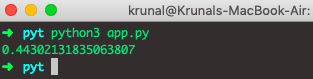We can also use random.randint() function to generate the random integer. See the below code example.

```# app.py

import random

print(random.randint(10, 100))```

The output of the above code is following.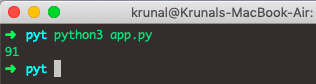## Floating Number using Python random module

We can generate the floating-point number using the Python random module.

See the following example.

```# app.py

from random import *
print(uniform(19, 21))```

See the output.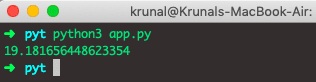## Python randrange() function

The following example generates a random number between 0 and 9.

See the below code.

```# app.py

from random import *
print("Random Integer: ",randrange(10, 22, 2))```

See the output.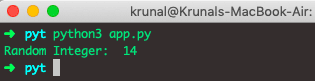## Randomly select an item from a List

Assume you have the following characters_list and you want to retrieve the item at random from this list. Let see how to do this.

```# app.py

import random
characters_list = ['Ironman', 'Captain America', 'Thor', 'Natasha', 'Hulk']
print("Select random element from characters_list: ", random.choice(characters_list))```

See the output.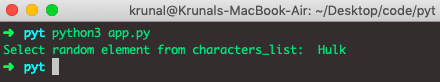### Python random.choice(seq)

We can use random.choice() method to pick the random element from a sequence.

Here sequence can be list or string. The method returns a single item from the sequence.

### Python random.sample(population, k)

Use the random.sample() method when we want to pick more multiple random elements from a population.

• The sample method returns the list of unique elements chosen from the population. Count of the total items depends on the size of the k.
• The population can be a list, set or any sequence.

See the following example code.

```# app.py

import random
list = [22,25,28,29,21]
print ("random.sample() ",random.sample(list,3))```

See the output.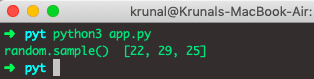## Python random.choices()

The syntax of random.choice() function is following.

`random.choices(population, weights=None, *, cum_weights=None, k=1)`

If you want to choose more than one element from the sequence randomly, then use the random.choices() method. The choices() method introduced in python version 3.6 and it can repeat the elements.

```# app.py

import random
list = [200, 300, 400, 500 ,600, 700, 800, 900]
sample = random.choices(list, k=3)
print("sampling with choices method ", sample)```

See the output.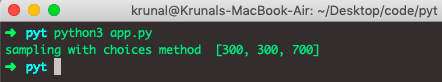### Python random.seed() Example

Python random.seed() method is used to initialize the pseudorandom number generator in Python.

Python random module uses the seed value as the base to generate the random number. If the seed value is not present, it takes the current system time. See the following syntax.

`random.seed(a=None, version=2)`

See the below code example.

```# app.py

import random
random.seed(4)
print("Random number with seed: ",random.random())```

See the output.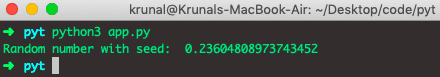### Python random.shuffle() Example

If we want to shuffle or randomize list or other sequence types. The shuffle function shuffles a list in-place. The most common example is the shuffle cards. See the below code example.

```# app.py

import random
list = [21,19,46,29,6]
random.shuffle(list)
print ("Shuffled list ", list)```

See the output.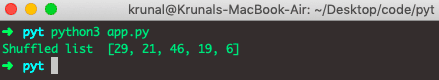Conclusively, Python Random Number Module Example is over.

This site uses Akismet to reduce spam. Learn how your comment data is processed.